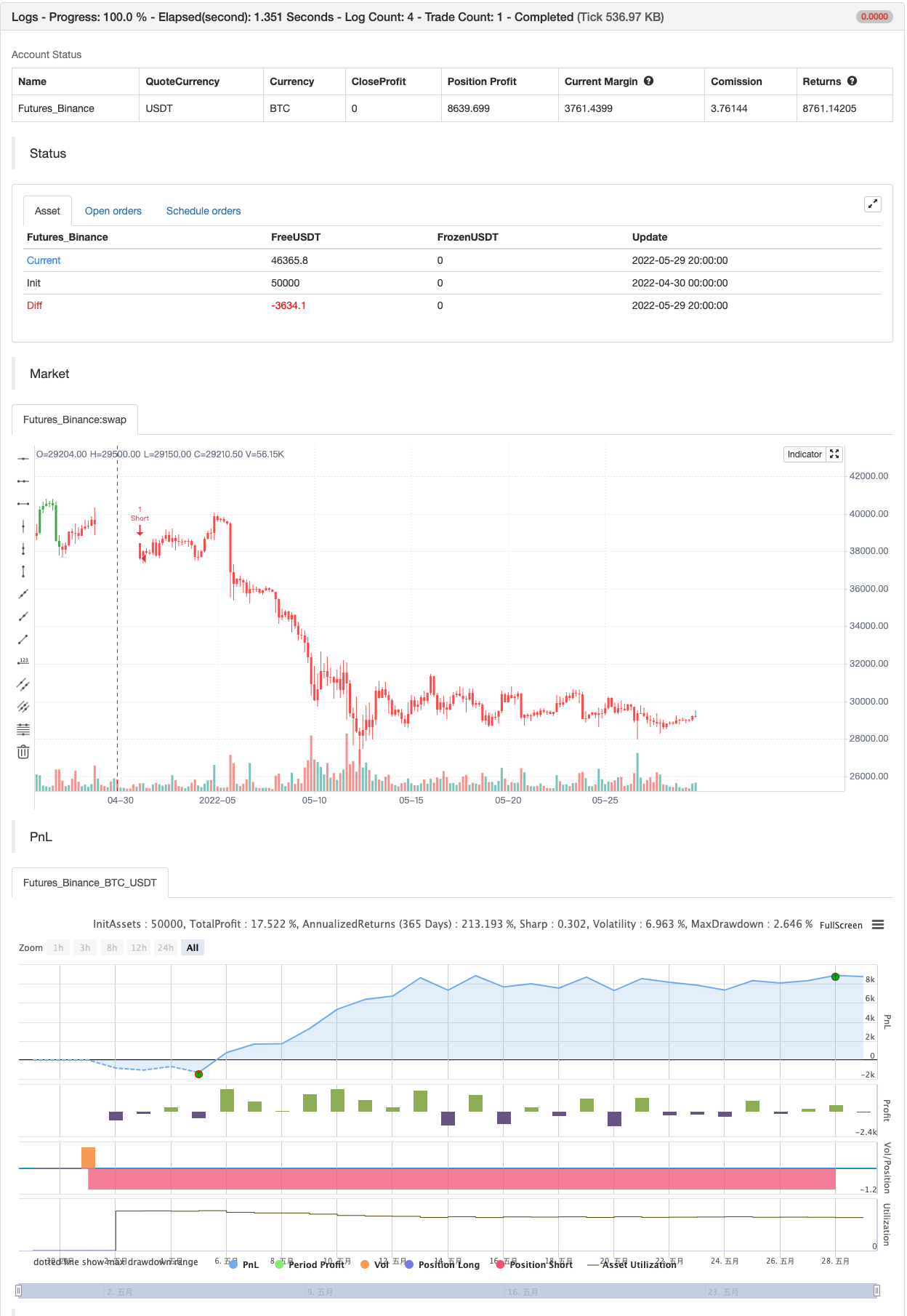# Support-Resistance breakout

Author: 张超, Date: 2022-05-31 18:20:45
Tags: SR

Strategy based on longing resistance breakout and shorting support breakout. It defines highs and lows using fractal with 2 bars for confirming high/lows. So it has 2 bars lag. It calculates the difference between sma with defined length (21 by default) of highs and of lows and uses it as alt SR level. This idea I took from synapticEx’s indicator Nebula-Advanced-Dynamic-Support-Resistance. Position enter is the breakout of SR, defined by fractals. Position exit is: bar change in opposite to position direction > difference is sma of highs and of lows.

backtest```/*backtest
start: 2022-04-30 00:00:00
end: 2022-05-29 23:59:00
period: 4h
basePeriod: 15m
exchanges: [{"eid":"Futures_Binance","currency":"BTC_USDT"}]
*/

//@version=4
strategy("SR TREND STRATEGY", shorttitle="SR TREND", overlay=true, calc_on_order_fills=true)
length = input(title="SR lookbak length", type=input.integer, defval=21)
h = bar_index>5 and high<high and high<high and high>high and high>high ? 1 : 0
l = bar_index>5 and low>low   and low>low   and low<low   and low<low   ? 1 : 0
ln = sum(l, length)
hn = sum(h, length)
hval =  h>0 ? high : 0
lval =  l>0 ? low  : 0
lsum = sum(lval, length)
hsum = sum(hval, length)
r = ln>0 and hn>0 ? abs((hsum/hn) - (lsum/ln)): 0
float lvalc = na
float lvalr = na
float hvalc = na
float hvalr = na
lvalc := lval and r>0 ? lval   : lvalc
lvalr := lval and r>0 ? lval+r : lvalr
hvalc := hval and r>0 ? hval   : hvalc
hvalr := hval and r>0 ? hval-r : hvalr
int trend=0
trend:=close > lvalr and close > hvalr ? 1 : close < lvalr and close < hvalr ? -1 : trend
strategy.close("Long", when=trend==-1)
strategy.close("Short", when=trend==1)
strategy.entry("Long", strategy.long, when=trend==1 and close>hvalc)
strategy.entry("Short", strategy.short, when=trend==-1 and close<lvalc)
int long=0
int short=0
long:= trend==1 and close>hvalc ? 1 : trend==-1 ? -1 : long
short:= trend==-1 and close<lvalc ? 1 : trend==1 ? -1 : short
barcolor(long>0? color.green : short>0? color.red : trend>0? color.white: trend<0 ? color.orange : color.blue)
```

Related

More